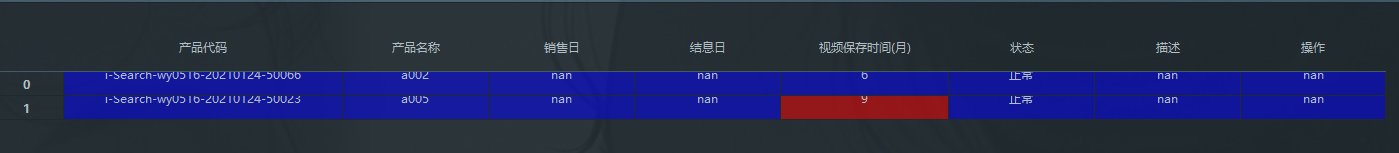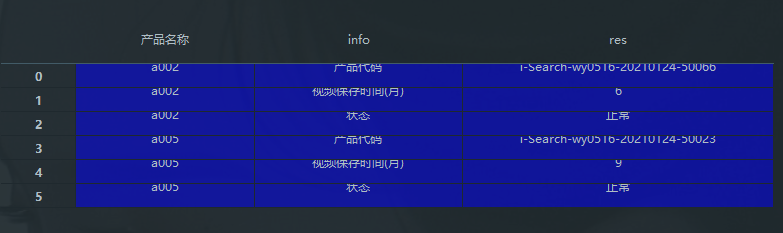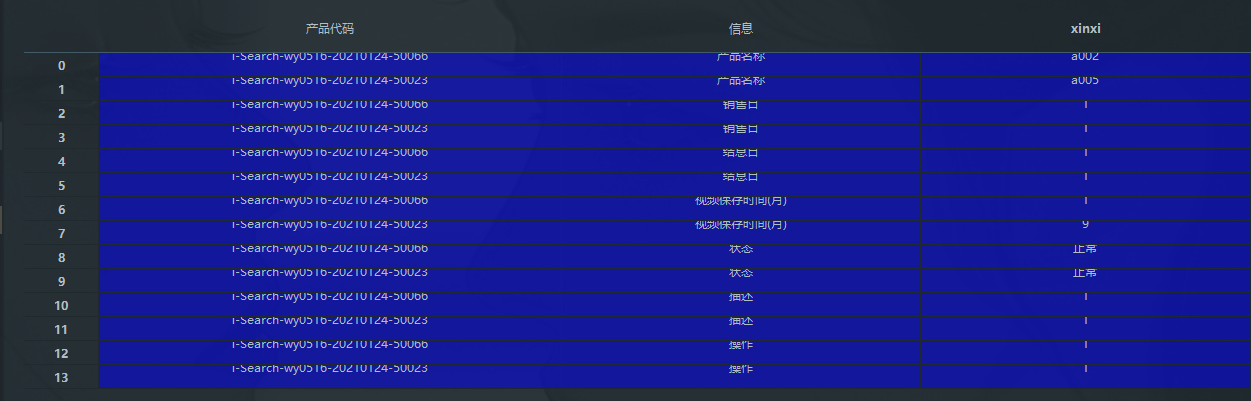# pandas 工具 excel 的行列转换处理学习笔记

import pandas as pd
import os
abs_path = os.path.abspath(‘.’)
file_path = os.path.join(abs_path,‘df_test.xlsx’)
aaa 如图：new_aaa = aaa.T
new_aaar 如图：df = aaa.set_index(‘产品名称’)
df = df.stack()
df.index = df.index.rename(‘info’,level=1)
df.name =‘res’
df = df.reset_index()
print(df)
df 如图：set_index 重新设置索引，stack()多级化索引，并将数据框（DataFrame）转换成序列（Series）。转置后，再用 reset_index() 将数据框还原成普通的二维表。

unstack 也是多级化索引，区别在于：stack 会把 None 自动过滤，unstack 保留 None 的数据

melt 也可以快速实现

`df2 = pd.melt(aaa,id_vars=['产品代码'],var_name = '信息',value_name='xinxi')`

df2 如图:`df2.sort_values(by=['产品代码'])`